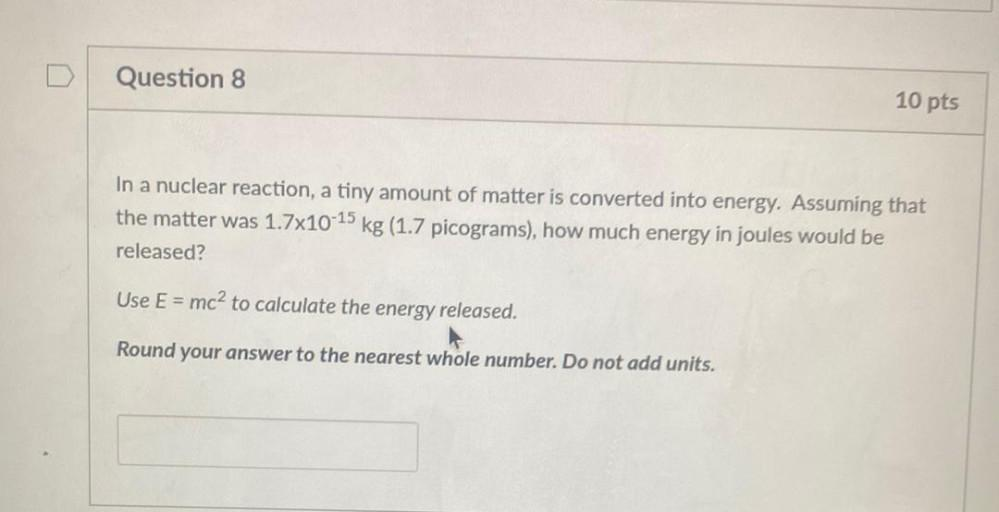Question:

# In a nuclear reaction, a tiny amount of matter is converted into energy. Assuming that the matter was 1.7x10-¹⁵ kg(1.7 picograms), how much energy in joules would be released? Use E = mc² to calculateIn a nuclear reaction, a tiny amount of matter is converted into energy. Assuming that the matter was 1.7x10-¹⁵ kg(1.7 picograms), how much energy in joules would be released? Use E = mc² to calculate the energy released.